Siegel domain

An unbounded domain in the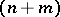-dimensional complex affine space of the form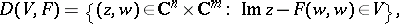whereis an open convex cone inand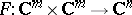is a mapping which is a-Hermitian form, i.e.is linear in the first argument,,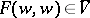(where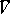is the closure of), andonly if. In the case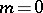(so that also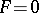) the domainis called a Siegel domain of the first kind and is simply denoted by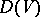; when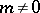,is called a Siegel domain of the second kind.

The simplest example of a Siegel domain (of the first kind) is the upper half-plane in. C.L. Siegel , in his studies on Abelian varieties, studied the domain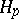in the space of complex symmetric matrices of order, formed by matrices with positive-definite imaginary part. This domain, now known as the Siegel upper half-plane (when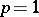it is the ordinary upper half-plane), is a Siegel domain of the first kind associated with the cone of positive-definite symmetric matrices of order. The general concept of a Siegel domain arose in connection with the theory of automorphic functions of several complex variables (see ). Subsequently this notion became central in the theory of homogeneous bounded domains (cf. Homogeneous bounded domain).

Any Siegel domain is analytically isomorphic to a bounded domain. For example, whena Siegel domain is isomorphic to the complex unit ball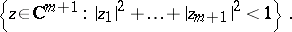Any homogeneous bounded domain is isomorphic to a Siegel domain which is homogeneous relative to affine transformations. Two Siegel domains are analytically isomorphic if and only if each can be transformed into the other by an affine transformation (see ).